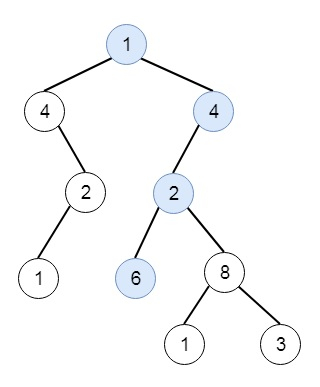# Linked List in Binary Tree in C++

C++Server Side ProgrammingProgramming

#### C in Depth: The Complete C Programming Guide for Beginners

45 Lectures 4.5 hours

#### Practical C++: Learn C++ Basics Step by Step

Most Popular

50 Lectures 4.5 hours

#### Master C and Embedded C Programming- Learn as you go

66 Lectures 5.5 hours

Suppose we have a binary tree root and a linked list with a head as the first node. We have to return True if all the elements in the linked list starting from the head correspond to some downward path connected in the binary tree otherwise False. So if the tree is like −And the linked list is [1,4,2,6], then the output will be true.

To solve this, we will follow these steps −

• Define a map dp

• Define a method called solve(), this will take head, root, and flag

• if the head is null, then return true, or if the root is null, return false

• if dp has a head, and dp[head] has the root and dp[head, root] has a flag, then return dp[head, root, flag]

• if the value of head = value of root, then

• ret := solve(next of head, left of the root, false) or solve(next of head, right of the root, false)

• if ret is set, then dp[head, root, flag] := true and return dp[head, root, flag]

• dp[head, root, flag] = solve(head, left of root, flag) OR solve(head, right of root, flag)

• return dp[head, root, flag]

• otherwise when flag is not set, then return dp[head, root, flag] := false

• otherwise return dp[head, root, flag] := solve(head, left of root, flag) or solve(head, right of root, flag)

• From the main method call solve(head, root, true)

## Example (C++)

Let us see the following implementation to get a better understanding −

Live Demo

#include <bits/stdc++.h>
using namespace std;
class ListNode{
public:
int val;
ListNode *next;
ListNode(int data){
val = data;
next = NULL;
}
};
ListNode *make_list(vector<int> v){
ListNode *head = new ListNode(v);
for(int i = 1; i<v.size(); i++){
ListNode *ptr = head;
while(ptr->next != NULL){
ptr = ptr->next;
}
ptr->next = new ListNode(v[i]);
}
}
class TreeNode{
public:
int val;
TreeNode *left, *right;
TreeNode(int data){
val = data;
left = right = NULL;
}
};
void insert(TreeNode **root, int val){
queue<TreeNode*> q;
q.push(*root);
while(q.size()){
TreeNode *temp = q.front();
q.pop();
if(!temp->left){
if(val != NULL)
temp->left = new TreeNode(val);
else
temp->left = new TreeNode(0);
return;
} else {
q.push(temp->left);
}
if(!temp->right){
if(val != NULL)
temp->right = new TreeNode(val);
else
temp->right = new TreeNode(0);
return;
} else {
q.push(temp->right);
}
}
}
TreeNode *make_tree(vector<int> v){
TreeNode *root = new TreeNode(v);
for(int i = 1; i<v.size(); i++){
insert(&root, v[i]);
}
return root;
}
class Solution {
public:
map < ListNode*, map<TreeNode*, map <bool, bool>> > dp;
bool solve(ListNode* head, TreeNode* root, bool flag = true){
if(head == NULL) return true;
if(!root) return false;
bool ret = solve(head->next, root->left, false) ||
if(ret) return dp[head][root][flag] = true;
flag) || solve(head, root->right, flag);
}else if(!flag) return dp[head][root][flag] = false;
else
flag) || solve(head, root->right, flag);
}
bool isSubPath(ListNode* head, TreeNode* root) {
}
};
main(){
vector<int> v = {1,4,2,6};
vector<int> v1 = {1,4,4,NULL,2,2,NULL,1,NULL,6,8,NULL,NULL,NULL,NULL,1,3};
ListNode *head = make_list(v);
TreeNode *root = make_tree(v1);
Solution ob;
cout << (ob.isSubPath(head, root));
}

## Input

[1,4,2,6]
[1,4,4,null,2,2,null,1,null,6,8,null,null,null,null,1,3]

## Output

1